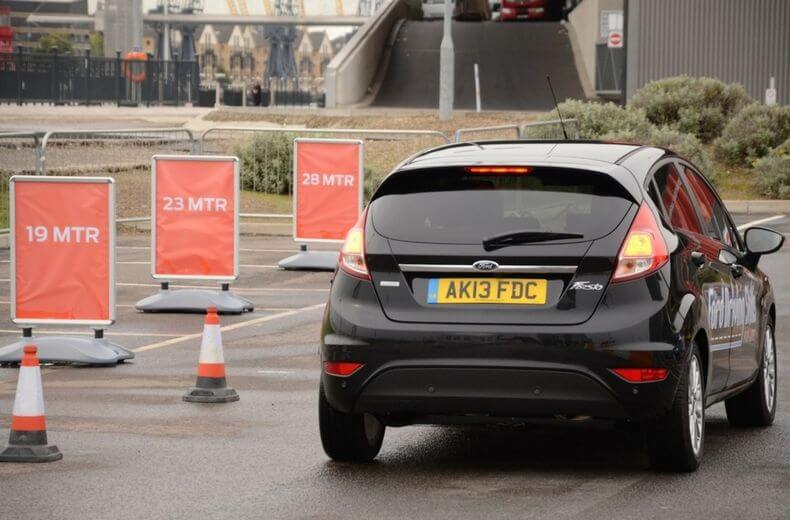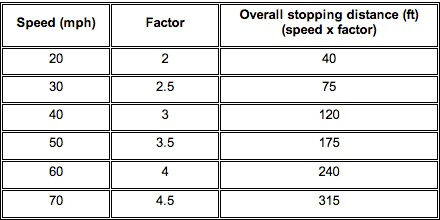# How to remember stopping distances for your theory test

A common question on the theory test is 'what is the [overall stopping, thinking or braking] distance at 30 mph?' etc.

Rather than remembering a list of the distances, you can calculate them instead using the method detailed below. It works for calculating the distances in feet. You can convert feet to metres afterwards if necessary.

No matter how well prepared you are for the UK driving theory test, you may be feeling a bit nervous about taking it. That's perfectly normal but excess nerves or anxiety can stop you performing at your best. So you need to know how to manage test nerves.

Overall Stopping Distance (on dry roads)

The overall stopping distance is built from the thinking distance, i.e. the distance the vehicle has travelled in the time taken to react to a hazard; and the braking distance, i.e. the distance travelled from the moment the brakes of the vehicle are applied to the point when the vehicle comes to a complete stop.

The table below applies a 'factor', dependent on the speed you are travelling, which is multiplied with the speed to calculate the overall stopping distance in feet.

The factors are easy to remember - just start at 2 for 20mph and add 0.5 for each 10 mph increase in speed.Example:

• Question: What is the overall stopping distance at 50mph?
• Answer: Factor for 50mph is 3.5 and so overall stopping distance at 50mph is 50 x 3.5 = 175 feet.

Thinking Distance

The thinking distance (in feet) is the same as the speed (in mph).

Example:

• Question: What is the thinking distance at 50mph?
• Answer: Thinking distance at 50mph is 50 feet.

Braking Distance

The braking distance is the overall stopping distance minus the thinking distance.

Example:

• Question: What is the braking distance at 50mph?
• Answer: Overall stopping distance at 50mph is 175 feet (see above).

Thinking distance at 50mph is 50 feet (see above). So braking distance is 175 - 50 = 125 feet.If you want to calculate stopping distances in metres instead of feet, simply do the calculations in feet and then convert the final answer back to metres:

1 foot = 0.3048 metres, or, to ease calculation, 1 foot is roughly equal to 3/10 metres, i.e. divide the distance by 10 and multiply by 3.

Example:

• Question: What is the overall stopping distance in metres distance at 40mph?
• Answer: Overall stopping distance at 40mph is 40 x 3 feet = 120 feet. 120 feet is approximately equal to 120 * (3/10) metres = (120/10)*3 metres = 12*3 metres = 36 metres.

Stopping distances on wet or icy roads

Remember in wet conditions stopping distances are doubled. On snow or icy roads stopping distances can be over 10 times that of normal dry conditions.